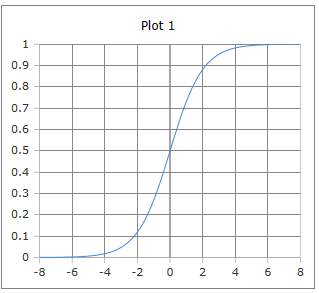# Sigmoid Function

Online calculator for calculating the sigmoid function

## Calculate the sigmoid function

Online calculator for calculating the Sigmoid function. The Sigmoid function refers to the function described in the formula below.

Calculator sigmoid function

 Input Argument Result Decimal places 0 1 2 3 4 6 8 10The curve of the sigmoid function

## Formulas for the sigmoid function

A sigmoid function, or S-function, is a mathematical function with an S-shaped graph. Here the sigmoid function is related to the special case of logistic function, which is described by the following equations.

$$\displaystyle sig(t)= \frac{1}{1+e^{-t}} = \frac{e^{t}}{1+e^{t}}=\frac{1}{2}·\left(1+tanh\frac{t}{2}\right)$$